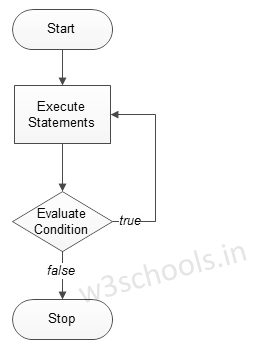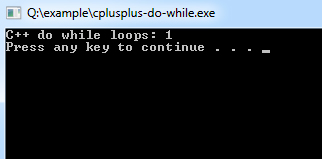##### C++ Programming Tutorial Index

C++ do while loops are very similar to the while loops, but it always executes the code block at least once and furthermore as long as the condition remains true. This is an exit-controlled loop.

The basic format of do while loop statement is:

Syntax:
``````do
{
statement(s);

}while( condition );``````

Figure - Flowchart of do while loop:### Example of a C++ Program to Demonstrate do while loop

Example:
``````#include <iostream>
using namespace std;

int main ()
{
/* local variable Initialization */
int n = 1,times=0;

/* do-while loops execution */
do
{
cout << "C++ do while loops: " << n <<endl;
n++;
}while( n <= times );
return 0;
}``````
Program Output: